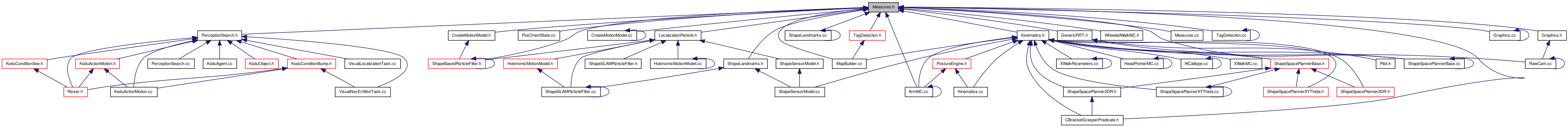Tekkotsu Homepage Demos Overview Downloads Dev. Resources Reference Credits

Measures.h File Reference

#include "Shared/fmatCore.h"
#include <cmath>
Include dependency graph for Measures.h:This graph shows which files directly or indirectly include this file:Go to the source code of this file.

Classes

class  PlaneEquation
Representation of a plane as ax + by + cz = d; used for representing the ground plane. More...
class  AngPi
Circular arithmetic on angles between 0 and pi (180 degrees). More...
class  AngTwoPi
Circular arithmetic on angles between 0 and two pi (360 degrees). More...
class  AngSignPi
Circular arithmetic on angles between -pi and pi (360 degrees). More...
class  AngSignTwoPi
Turn with an explicit direction: angles between -2*pi and 2*pi (+/- 360 degrees). More...

Typedefs

typedef float coordinate_t
type used for positional coordinates
typedef float orientation_t
type used for orientation values (0 to Pi)
typedef float direction_t
type used for direction values (0 to 2*Pi)
typedef coordinate_t Slope
type used for ratio of coordinate offsets

Functions

std::ostream & operator<< (std::ostream &os, const PlaneEquation &p)
AngPi angdist (AngPi const &arg1, AngPi const &arg2)
Angular distance: value is between 0 and pi/2.
AngPi angdist (AngTwoPi const &arg1, AngTwoPi const &arg2)
Angular distance: value is between 0 and pi.
AngPi angdist (AngSignPi const &arg1, AngSignPi const &arg2)
Angular distance: value is between 0 and pi.

Variables

const direction_t Pi = static_cast<direction_t>(M_PI)
shorthand for M_PI from math.h
const direction_t TwoPi = static_cast<direction_t>(2*M_PI)
shorthand for 2*M_PI
const Slope BIG_SLOPE = static_cast<Slope>(5000)
slopes larger than this are considered vertical, or in other words, infinite slopes are rounded to this

Typedef Documentation

 typedef float coordinate_t

type used for positional coordinates

Definition at line 34 of file Measures.h.

 typedef float direction_t

type used for direction values (0 to 2*Pi)

Definition at line 36 of file Measures.h.

 typedef float orientation_t

type used for orientation values (0 to Pi)

Definition at line 35 of file Measures.h.

 typedef coordinate_t Slope

type used for ratio of coordinate offsets

Definition at line 41 of file Measures.h.

Function Documentation

 AngPi angdist ( AngSignPi const & arg1, AngSignPi const & arg2 )

Angular distance: value is between 0 and pi.

Definition at line 93 of file Measures.cc.

 AngPi angdist ( AngTwoPi const & arg1, AngTwoPi const & arg2 )

Angular distance: value is between 0 and pi.

Definition at line 66 of file Measures.cc.

 AngPi angdist ( AngPi const & arg1, AngPi const & arg2 )

Angular distance: value is between 0 and pi/2.

Definition at line 41 of file Measures.cc.

 std::ostream& operator<< ( std::ostream & os, const PlaneEquation & p )

Definition at line 15 of file Measures.cc.

Variable Documentation

 const Slope BIG_SLOPE = static_cast(5000)

slopes larger than this are considered vertical, or in other words, infinite slopes are rounded to this

Definition at line 42 of file Measures.h.

 const direction_t Pi = static_cast(M_PI)

shorthand for M_PI from math.h

Definition at line 38 of file Measures.h.

 const direction_t TwoPi = static_cast(2*M_PI)

shorthand for 2*M_PI

Definition at line 39 of file Measures.h.

 Tekkotsu v5.1CVS Generated Mon May 9 04:58:59 2016 by Doxygen 1.6.3